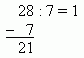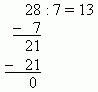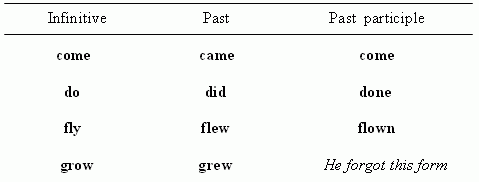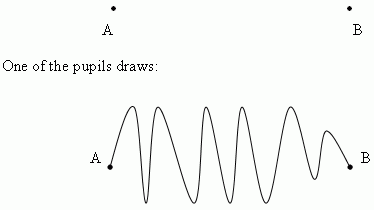M a t h e m a t i c a l   j o k e s

How old were they ?

Two students-mathematicians, having birthdays on the same day, wished each other many happy returns on this day.  One of them said:
- You’ll have such birthday only in 11 years.
- Okay, but you’ll have such birthday only in 96 years.
Both of them were satisfied with each other. How old were they on this day ?

S o l u t i o n.   One of them was 25, and other 24.  Why?
25=5 2 , the next square is 6 2 =36, i.e. in 11 years.
24=4!, the next factorial is 5!=120,  i.e. in 96 years.

New laws of arithmetic

A pupil divides 28 by 7 and receives as a result 13. His teacher asks him:
- How did you receive this result ?
- First I divide 8 by 7 and receive 1. Now 1 x 7 = 7 and I write:Now I divide 21 by 7 and receive 3. Now I write:The teacher asks him to check the result by multiplication. The pupil answers:
- I execute 13 x 7  by the steps:
1)   1 x 7 = 7,
2)   3 x 7 = 21,
3)   7 + 21 = 28.

About application of mathematics in linguistics

A teacher of English was ill and a teacher of mathematics replaced him. He began to compose a table of irregular verbs:Then he said:
- Okay, I mark this form as x . Then it’s possible to compose the proportion:Why do we hear a clatter of train wheels ?

Answer: Wheel has a form of a circle. An area of a circle is equal to p r 2 , so this square clatters.

What difference between physicist and programmer ?

Answer: Physicist thinks that 1 kilobyte = 1000 bytes, and programmer thinks that 1 kilometer = 1024 meters.

The shortest distance between two points

At a geometry lesson a teacher asks to represent the shortest distance between the two points A and B on a blackboard: